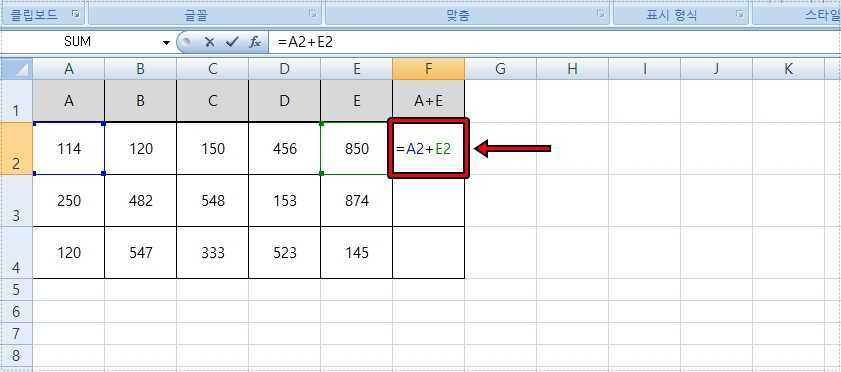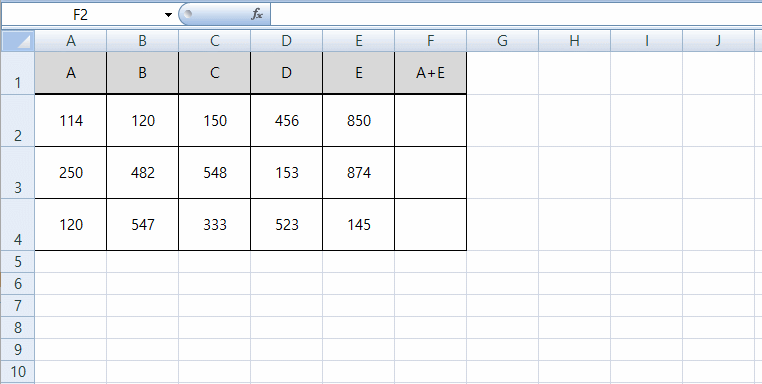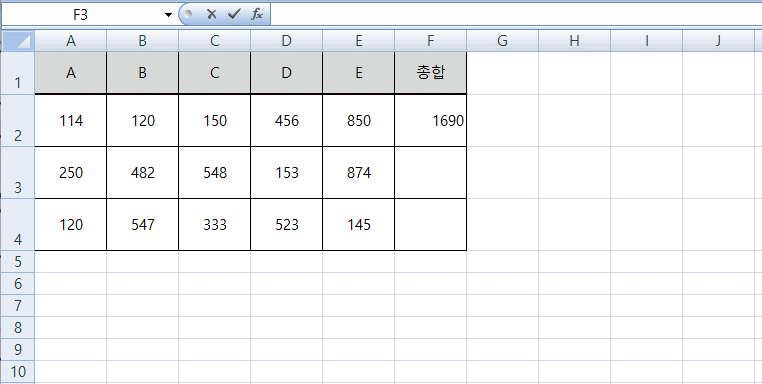# How to add Excel and use SUM function

Excel is a program that is used a lot in office work because it can make documents from ordinary documents to calculations, and it is difficult to use functions. So, starting with the add Excel function, I want to learn how to use Excel from various functions.

To add, you need to know how to use the SUM function, but you don’t have to memorize all functions because Excel is a function wizard that helps you use them. Let’s take an example of how to use the SUM function.

#### 1. Add Excel Cell unitsTo add in cells, enter the corresponding cell coordinates after = and enter + in the middle to add cells. If you want to add these cells, you can type =A2+B2+C2+D2+E2.If you have multiple functions, it can be difficult to enter cells. If that happens, you can select a cell after entering =, hold down the Ctrl key, and select a different cell, then you can select multiple cells. To buy more, you must select and enter + separately.If you enter = in the sum cell of the documents to be calculated, you can create a function, even in the function input above. If you type =SUM, you get a similar list of functions, so even if you don’t remember the function, you can find it by entering something similar.

The function to add the total from A2 to E2 is to add the specified cells when you enter the cells starting with =SUM (A2:E2) and then the last cell is entered after adding a paired point (:).

Pair points (:) represent the interval, which means to add A2 to E2.

A comba(,) is a cell-by-cell input, for example =SUM(A2,E2), which means adding A2 and E2.Because it’s difficult to enter a cell directly, after you type =SUM, you drag the cells you want to add with your mouse, and that cell is automatically entered.

#### 3. Using the Function WizardWhen you cannot remember a function, press the fx button at the top and the Function Wizard window will appear. If you enter sum, subtraction, and so on in the function search, you can find the function and select the function you found.When you select the SUM function that can be add Excel, the Function Arguments window appears, where you select the cells to add with the mouse, and you can see the additional values. The function wizard is convenient if you do not understand the function because it also provides a user description.

You can choose from the three options above to add Excel file, and since the function wizard can see all the available functions, one by one, it will help you learn the function.

▶ Replace excel row with columns To use the Paste option

▶ Display specific values using Excel conditional formatting

▶ How to insert and attach Excel PDF file contents

Scroll to Top